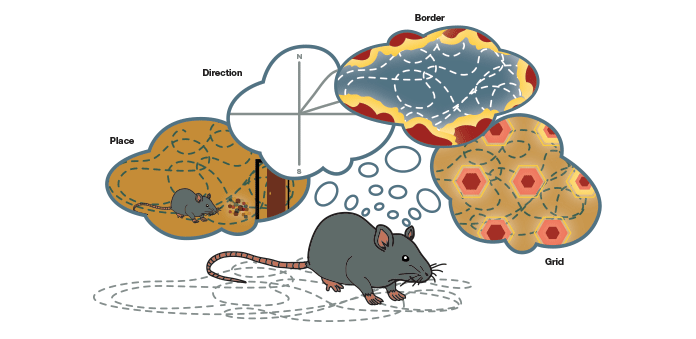Successor Representations

Successor representations were introduced by Dayan in 1993, as a way to represent states by thinking of how “similarity” for TD learning is similar to the temporal sequence of states that can be reached from a given state.

Dayan derived it in the tabular case, but let’s do it when assuming a feature vector $\phi$.

We assumes that the reward function can be factorised linearly: $$r(s) = \phi(s) \cdot w$$

This can then be inserted back into the value expectation formula: $$\begin{array}{rl} Q^\pi(s, a) &= E \left\lbrack \sum_{t=0}^\infty \gamma^t R(s_t) ~|~ s_o = s, a_0 = a \right\rbrack \\ & = E\left\lbrack \sum_t^\infty \gamma^t \phi(s_t) \cdot w ~|~ s_0=s, a_0 = a \right\rbrack \\ & = E\left\lbrack \sum_t^\infty \gamma^t \phi(s_t) ~|~ s_0=s, a_0 = a \right\rbrack \cdot w \\ & = \psi^\pi(s, a) \cdot w \end{array}$$

$\psi^\pi$ represents the Successor features, and does not depend on the rewards. It also represents a partial model of the world / occupancy of the states given the behaviour policy.

$w$ represents the rewards or the goal, and can be thought of as preferences about the given features $\phi(s)$ in a given task.

Assuming that the features $\phi(s)$ are good and consistent across a domain, we can imagine transfering to new tasks really quickly, as we simply need to learn new goal vectors $w$.

In Dayan’s case, the features $\phi(s)$ were directly the one-hot occupancy of a tabular state-space. This means that the successor features directly corresponds to the visitation counts under a policy. This is not as simple in more complex scenarios or when we want to learn $\phi$.

One important observation, also mentioned in passing by Dayan, is that one can rewrite the definition of the successor features $\psi$ using the Value expectation formula, obtaining independent Bellman updates for every components of $\phi$:

$$E\left\lbrack \sum_t^\infty \gamma^t \phi_i(s_t) ~|~ s_0=s, a_0 = a \right\rbrack$$

$$= E\left\lbrack \phi_i(s_t) + \gamma \psi_i(s_{t+1}, a^\star) - \psi_i(s_t, a_t) ~|~ s_0=s, a_0 = a \right\rbrack$$

In other terms, the features $\phi(s)$ behave as the rewards in a Bellman update, where $\psi(s, a)$ takes the role of the Q-values.

• Peter Dayan, “Improving generalization for temporal difference learning: The successor representation”, Neural Computation, 5(4):613–624, 1993. pdf